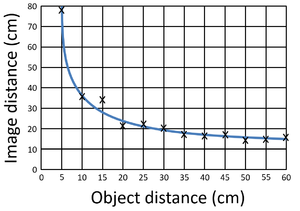## Key Stage 3

### Meaning

Gradient is how steep or shallow a line is compared to the horizontal.

The gradient of a slope is how much the height increases as the horizontal distance increases. A steep slope has a large increase in height over a short horizontal distance.
The gradient on a scatter graph is the rate at which the variable on the y-axis changes with a change on the x-axis.
A positive gradient on a scatter graph is one where as x increases, y increases.
A negative gradient on a scatter graph in one where as x increases, y decreases.

### Equation

Gradient = (Change in y)/(Change in x)

$$m=\frac{y_2-y_1}{x_2-x_1}$$

Where

$$m$$ = The gradient.

$$y_2$$ = The final y value.

$$y_1$$ = The initial y value.

$$x_2$$ = The final x value.

$$x_2$$ = The initial x value.

## Key Stage 4

### Meaning

Gradient is how steep or shallow a line is compared to the horizontal.

The gradient of a slope is how much the height increases as the horizontal distance increases. A steep slope has a large increase in height over a short horizontal distance.
The gradient on a scatter graph is the rate at which the variable on the y-axis changes with a change on the x-axis.
A positive gradient on a scatter graph is one where as x increases, y increases.
A negative gradient on a scatter graph in one where as x increases, y decreases.

### Equation

Gradient = (Change in y)/(Change in x)

$$m=\frac{y_2-y_1}{x_2-x_1}$$

Where

$$m$$ = The gradient.

$$y_2$$ = The final y value.

$$y_1$$ = The initial y value.

$$x_2$$ = The final x value.

$$x_2$$ = The initial x value.This scatter graph of Image Distance against Object Distance of a Lens begins with a steep negative gradient which becomes more shallow until the gradient is almost zero. This scatter graph showing how temperature affects enzyme activity begins with a steep positive gradient but then changes to a steep negative gradient.